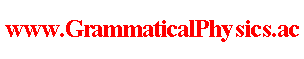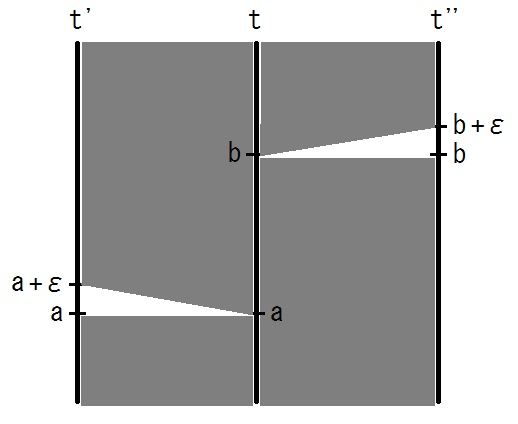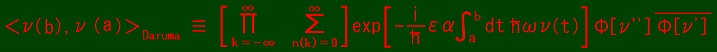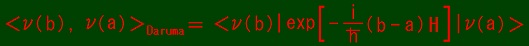since 2006
Help　Sitemap
< Forum >
< Products >
< Quantum History Theory >
< Stacked Daruma Game Formula >

In this page, I make Stacked Daruma Game Formula by using Exact Solution of Uda Equation (5).
Stacked Daruma Formula gives transition amplitude.

First I chose a quantum history represented by functional Φ such that
Φ[ν] ＝ ∑k exp{α∫-∞dt[-iωtν(t) + a(k)ν(t) + ib(k)ν(t)]}
where a(k)n and b(k)n are defined as follows.
|uk＞ = ∑n=0 λ(k)n|n＞ = ∑n=0 exp(a(k)n + ib(k)n)|n＞.
{ |uk＞ | ∃k } is a orthonotmal basis of the state space.

 ν'(t') = ν(t') if t' ＜ a. ν'(t') = ν(a) if a ≦ t' ＜ a + ε. ν'(t') = ν(t' - ε) if t' ≧ a + ε. ν''(t'') = ν(t'') if t'' ＜ b. ν''(t'') = ν(b) if b ≦ t'' ＜ b + ε. ν''(t'') = ν(t'' - ε) if t'' ≧ b + ε.ε ＝ 1/α, b - a ＝ Nε,
ν(t) ＝ nk if a + kε ≦ t ＜ a + (k + 1)ε..

Φ[ν''] = ∑k exp{α∫-∞bdt''[-iωt''ν''(t'') + a(k)ν''(t'') + ib(k)ν''(t'')]
+ α∫bb+εdt''[-iωt''ν''(t'') + a(k)ν''(t'') + ib(k)ν''(t'')]
+ α∫b+εdt''[-iωt''ν''(t'') + a(k)ν''(t'') + ib(k)ν''(t'')]}
= ∑k exp{α∫-∞bdt''[-iωt''ν(t'') + a(k)ν(t'') + ib(k)ν(t'')]
+ α∫bb+εdt''[-iωt''ν(b) + a(k)ν(b) + ib(k)ν(b)]
+ α∫b+εdt''[-iωt''ν(t'' - ε) + a(k)ν(t''-ε) + ib(k)ν(t''-ε)]}
= ∑k exp{αε[-iω(b + ε/2)ν(b) + a(k)ν(b) + ib(k)ν(b)]}
× exp{α∫-∞bdt[-iωtν(t) + a(k)ν(t) + ib(k)ν(t)] + α∫bdt[-iω(t + ε)ν(t) + a(k)ν(t) + ib(k)ν(t)]}
= ∑k ψk(ν(b), b + ε/2) exp{α∫-∞dt[-iωtν(t) + a(k)ν(t) + ib(k)ν(t)] - iωεα∫bdt ν(t)}.

Φ[ν']* = ∑j ψj(ν(a), a + ε/2)* exp{α∫-∞dt[iωtν(t) + a(j)ν(t) - ib(j)ν(t)] + iωεα∫adt ν(t)}.

Φ[ν''] Φ[ν']* = ∑kj ψk(ν(b), b + ε/2)ψj(ν(a), a + ε/2)*
× exp{α∫-∞dt[a(k)ν(t) + ib(k)ν(t)] exp{α∫-∞dt[a(j)ν(t) - ib(j)ν(t)]
× exp[iωεα∫abdt ν(t)]
= ∑kj ψk(ν(b), b + ε/2)ψj(ν(a), a + ε/2)*s=-∞ λ(k)n(s) λ(j)n(s)*] exp[(i/h)εα∫abdt hων(t)].

＜ν(b), ν(a)＞Daruma = ∑kj ψk(ν(b), b + ε/2)ψj(ν(a), a + ε/2)*jk)
= ∑k ψk(ν(b), b + ε/2)ψk(ν(a), a + ε/2)*　∵ (δjk) = δjk
= ∑k ＜ν(b)|exp[-(i/h)(b + ε/2)H]|uk＞＜uk|exp[(i/h)(a + ε/2)H]|ν(a)＞
= ＜ν(b)| exp[-(i/h)(b + ε/2)H] exp[(i/h)(a + ε/2)H] |ν(a)＞
= ＜ν(b)| exp[-(i/h)(b - a)H] |ν(a)＞.= exp[-(i/h)(b - a)hων(a)]δν(b)ν(a)
= exp[-iω(b - a)ν(a)]δν(b)ν(a).

Perhaps Daruma contraction can be defined as
＜χ(b), χ(a)＞Daruma = ∑ν(b)=0ν(a)=0 ＜vχ(b)|ν(b)＞＜ν(b), ν(a)＞Daruma＜ν(a)|vχ(a)
= ∑ν(b)=0ν(a)=0 ＜vχ(b)|ν(b)＞＜ν(b)| exp[-(i/h)(b - a)H] |ν(a)＞＜ν(a)|vχ(a)
= ＜vχ(b)| exp[-(i/h)(b - a)H] |vχ(a)
where |vx＞ is an eigenvector of the position operator with eigenvalue x.

For example, if we take ε = 1/(2α),
k=-∞n(k)=0] Πs=-∞(k)n(s)]1/2(j)n(s)*]1/2
arises as a factor in the calculation.
However, ∑n=0(k)n]1/2(j)n*]1/2 is not concerned with ∑n=0λ(k)n λ(j)n* = δjk at all.
So, ε ≠ 1/α is impossible.
Especially ε → +0 is impossible because ∑n=0(k)n]αε(j)n*]αε → ∑n=0 1 = ∞ as ε → +0.

If λ(k)n = δkn and ε → +0,
n=0(k)n]αε(j)n*]αε = ∑n=0 δknδjn = δjk,
exp{αε[-iω(b + ε/2)ν(b) + a(k)ν(b) + ib(k)ν(b)]} = [ψk(ν(b), b + ε/2)]αε = δν(b)kexp[-ikωαε(b + ε/2)] → δν(b)k,
＜ν(b), ν(a)＞Daruma = ∑kj δν(b)kδν(a)jjk)
= ∑k δν(b)kδν(a)k
= δν(b)ν(a)
＝ ＜ν(b)|ν(a)＞.
This result also is undesirable because it does not represent time development at all.

Can ＜ν(b), ν(a)＞Daruma be modified as
＜ν(b), ν(a)＞Daruma = [Πk=-∞n(k)=0]exp[-(i/h)∫abdt hων(t)] {Φ[ν''] Φ[ν']*}1/(εα)
and ε → +0 ?
Such a modification is also impossible because Φ[ν] consists of many terms.
Each term of Φ[ν''] Φ[ν']* is not raised to the αε-th power.

If λ(k)n = δkn and ε → +0 and
＜ν(b), ν(a)＞Daruma = {[Πk=-∞n(k)=0]exp[-(i/h)εα∫abdt hων(t)]Φ[ν''] Φ[ν']*}1/(εα),
＜ν(b), ν(a)＞Daruma = δν(b)ν(a)exp[-iω(b - a)ν(a)] = ＜ν(b)| exp[-(i/h)(b - a)H] |ν(a)＞.
This might be another candidate but I feel that this too is not very plausible because of λ(k)n = δkn and
exp[-(i/h)εα∫abdt hων(t)].

So, now I rechose a quantum history represented by functional Φ such that
Φ[ν] ＝ ∑rk exp{α∫-∞dt[-iωtν(t) + a(r, k)ν(t) + ib(r, k)ν(t)]}
where a(r, k)n and b(r, k)n are defined as follows.
|u(r)k＞ = ∑n=0 λ(r, k)n|n＞ = ∑n=0 exp(a(r, k)n + ib(r, k)n)|n＞.
Br ≡ { |u(r)k＞ | ∃k } is an orthonotmal basis of the state space for each r.
∀r; ∀k; ∀s; r ≠ s ⇒ not[∃j; ∃c ∈ C; |u(r)k＞ = c|u(s)j＞].

n=0 λ(r, k)n λ(s, j)n* = ∑n=0 ＜n|u(r)k＞ ＜u(s)j|n＞ = ＜u(s)j|u(r)k＞.
r ≠ s ⇒ ∀j; ∀k; | ＜u(s)j|u(r)k＞ | ＜ 1.
∴ [∑n=0 λ(r, k)n λ(s, j)n*] = δrsδjk.

＜ν(b), ν(a)＞Daruma = (∑r 1) × ＜ν(b)| exp[-(i/h)(b - a)H] |ν(a)＞.

---